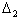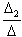Methods of solving linear systems - Part 2

Cramer's method is another way to solve a system of linear equations

The first step is to calculate the determinant, as before

We then REPLACE the first column of the coefficient matrix with the constant vector and calculate the determinant for that..... say... the first variable is solved asWe REPLACE the second column of the coefficient matrix with the constant vector and again calculate a determinant .... call it.... and the second variable is solved asWe repeat the pattern for the third variable... and we're done... assuming a 3 x 3 matrix.

You can see our WORKED EXAMPLE........ HEREEL10A Homepage